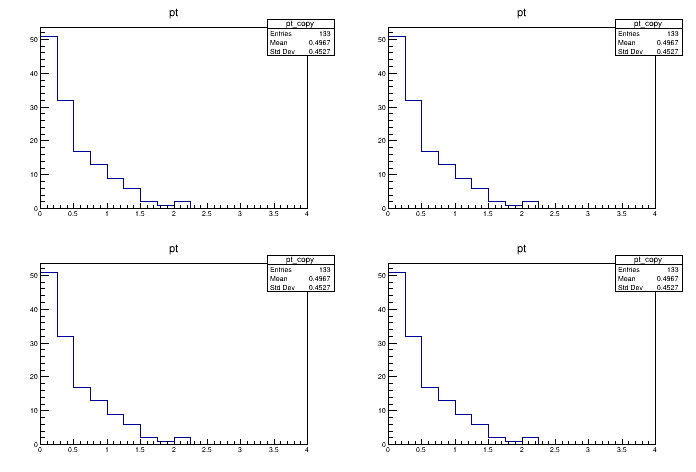# Df 0 1 7_Vec Ops H E P¶

Use RVecs to plot the transverse momentum of selected particles.

This tutorial shows how VecOps can be used to slim down the programming model typically adopted in HEP for analysis. In this case we have a dataset containing the kinematic properties of particles stored in individual arrays. We want to plot the transverse momentum of these particles if the energy is greater than 100.

Author: Danilo Piparo (CERN), Andre Vieira Silva
This notebook tutorial was automatically generated with ROOTBOOK-izer from the macro found in the ROOT repository on Tuesday, June 15, 2021 at 07:18 AM.

In :
auto filename = gROOT->GetTutorialDir() + "/dataframe/df017_vecOpsHEP.root";
auto treename = "myDataset";
using doubles = ROOT::VecOps::RVec<double>;
using RDF = ROOT::RDataFrame;


A helper function is created:

In :
%%cpp -d
void WithTTreeReader()
{
TFile f(filename);
TTreeReader tr(treename, &f);
TTreeReaderArray<double> px(tr, "px");
TTreeReaderArray<double> py(tr, "py");
TTreeReaderArray<double> E(tr, "E");

TH1F h("pt", "pt", 16, 0, 4);

while (tr.Next()) {
for (auto i=0U;i < px.GetSize(); ++i) {
if (E[i] > 100) h.Fill(sqrt(px[i]*px[i] + py[i]*py[i]));
}
}
h.DrawCopy();
}


A helper function is created:

In :
%%cpp -d
void WithRDataFrame()
{
RDF f(treename, filename.Data());
auto CalcPt = [](doubles &px, doubles &py, doubles &E) {
doubles v;
for (auto i=0U;i < px.size(); ++i) {
if (E[i] > 100) {
v.emplace_back(sqrt(px[i]*px[i] + py[i]*py[i]));
}
}
return v;
};
f.Define("pt", CalcPt, {"px", "py", "E"})
.Histo1D<doubles>({"pt", "pt", 16, 0, 4}, "pt")->DrawCopy();
}


A helper function is created:

In :
%%cpp -d
void WithRDataFrameVecOps()
{
RDF f(treename, filename.Data());
auto CalcPt = [](doubles &px, doubles &py, doubles &E) {
auto pt = sqrt(px*px + py*py);
return pt[E>100];
};
f.Define("good_pt", CalcPt, {"px", "py", "E"})
.Histo1D<doubles>({"pt", "pt", 16, 0, 4}, "good_pt")->DrawCopy();
}


A helper function is created:

In :
%%cpp -d
void WithRDataFrameVecOpsJit()
{
RDF f(treename, filename.Data());
f.Define("good_pt", "sqrt(px*px + py*py)[E>100]")
.Histo1D({"pt", "pt", 16, 0, 4}, "good_pt")->DrawCopy();
}


We plot four times the same quantity, the key is to look into the implementation of the functions above

In :
auto c = new TCanvas();
c->Divide(2,2);
c->cd(1);
WithTTreeReader();
c->cd(2);
WithRDataFrame();
c->cd(3);
WithRDataFrameVecOps();
c->cd(4);
WithRDataFrameVecOpsJit();


Draw all canvases

In :
gROOT->GetListOfCanvases()->Draw()